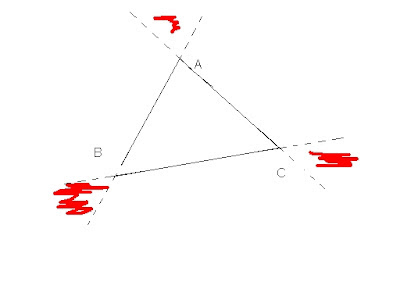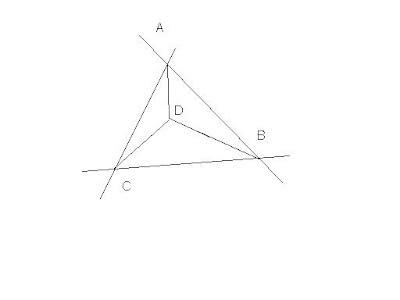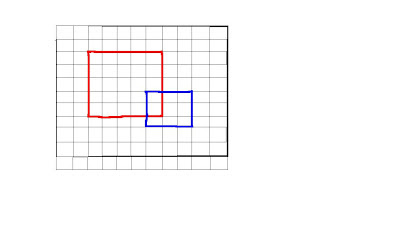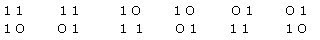## Monday, December 03, 2007

### Goodbye math blog

To whomsoever it may concern,

I have finally decided to stop writing this math blog. This has no bearing on rest of the contributors. It has been a great journey, I see myself today with a lot more mathematical maturity as I go through old to new posts. The reason to stop is not because I am getting absorbed into more serious math but the lack of purpose for which the blog was created nearly two years ago. ( I feel it is the time to tell the story, how the blog started) I had started messaging friends some curious problems that I normally kept creating for fun, later Sudhir suggested 'why don't you blog?' as it reaches people and people can comment. From then on I have posting problems mostly recreational, though I agree that my recent posts were a bit serious.

But these days there has been no response, comments like before and therefor feel the blog does not serve the purpose it was created for. I did or do not expect that I get comments that are mathematical, but some 'tries' as many of them are reachable to a layman, neither I feel like writing about what problems I am thinking about. So

Yours,
Srikanth

## Thursday, November 22, 2007

### New books in mathematics

You can find a lot of free e-books here.Books range from math classics to recently released ones gracefully violating copyrights.I simply believe knowledge should be free.

Some good books of my interest:

1.The art of Counting - Paul Erdos.

2.Combinatorics - Peter Cameron.

3.A course in combinatorics - van Lint and Wilson

4.Enumerative Combinatorics - R P Stanley

5.Research Problems in Discrete geometry - Peter Brass et al

## Tuesday, November 20, 2007

### klien's problem

I found this problem asing to be attacked by PHP, later I learnt this was generalized by Erdos and Szekeres(happy ending problem).

Let there be 5 points arbitrarily lying on a plane.Prove that we can find four points that form a convex quadrilateral.

classic proof: If the smallest convex polygon encompassing all the points is quadrilateral or pentagon, then we are done. Else, if it is a triangle (say ABC) and D and E are interior points.Among three points A, B, C two points lie on one side of DE and we are done.
Another proof:(I feel this is not elegant as the previous one)

Let three points A,B,C form a triangle.Now I think how I can introduce the 4th point.Reffering to pic1 if I introduce the 4th point in the non red regions we are done. So let me proceed by putting 4th point in red region. This results in pic2 that is equivalent to introducing the 4th point within triangle ABC.If the 5th point is introduced outside triangle ABC we are done.Else if introduced within ABC we get a convex quadrilateral involving D.## Monday, November 19, 2007

### Hit by PHP (Pigeon hole principle)

Problem: For coprime a and b, a/b has period of reccurance of length atmost b-1.

This next one is a classic.
Let there be n integers not necessarily distinct.Prove that we pick k (< (n+1)) integers which is divisible by n.

Let i_1, i_2,..., i_n be the integers.

Let a_1=i_1,
a_2=i_1 + i_2
.
.
a_n=i_1+..+i_n

If one of the a_j ( j=1,..,n ) is divisible by n we are done,else by php we can find a_r and a_s (r>s)giving same remainder. Then a_r-a_s gives rem 0 and we are done.

## Wednesday, November 14, 2007

### Yet another counting problemThis seems to fall for computation, I am looking at a possible elegant solution.
Problem:Let us have a n*n square.Two squares(both of dimension less than n*n)form an unordered pair if

(i) They have atleast one 1*1 square common.

(ii)One is not contained in the other.

Compute the number of unordered pairs.

(nothing sacred about squares, rectangles must make the problem tougher)

## Friday, November 02, 2007

### Computing a few Invertible matrices

Problem: Enumerate and generate the number of invertible matrices (n*n) with each entry either 0 or 1.

Example: For n=2, we have a set of 6 matrices. They areInterestingly, these matrices form a group under matrix multiplication.

A much more harder problem would be to enumerate and generate all invertible (n*n) matrices with enrites fron the set { 0,1,...,n-1 }.

## Tuesday, October 30, 2007

### Linear Independence

This problem is the generalization of exercise 2.3.9 from 'linear algebra' Hoffman and Kunze.

Let the set of vectorsbe linearly independent. Prove thatare also linearly independent givenI meanand so on.

ps: I proved it by proving a certain matrix to be invertible and that was pretty cumbersome. Hoping for a better pretty solution :)